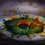# An awesome generalisation- of Bashing Unavailable

If you were given the three equations $\color{Blue}{a+b+c=1}$$\color{Blue}{a^2+b^2+c^2=2}$ $\color{Blue}{a^3+b^3+c^3=3}$

And then the values of $\color{#D61F06}{a^n+b^n+c^n}$ are asked for some $n \in \mathbb{Z}^+$, then you can get the answer by long expansions, squaring, getting needed terms etc, but there is a smart way too !

Some days ago, i had shared a set by name Bashing Unavailable...Awesome problems , which was on problems of this type , for $n \in \{ 4,5,6,7,8,9,10\}$.

If you haven't tried the set, I prefer telling you to try it before you read this note.

You can get the thing for $n=4$ by algebraic manipulations (and also for further values of $n$ ) , for example this "part of the solution" which I knowingly wrote to the first problem of the set.img

This way, you can get the values.... but here goes the smarter way, the $\color{#69047E}{\textbf{generalisation}}$. My way is similar to my friend Aamir Faisal Ansari,a worth following person's way...

See that as shown in the image, we can get values of $ab+bc+ca=\dfrac{-1}{2}$ and $abc=\dfrac{1}{6}$

Next, let's define a sequence $\{t_n\}$ as $t_n=a^n+b^n+c^n$

Then, we do the following algebraic manipulation which will give us the generalisation

$a^n+b^n+c^n=(a+b+c)(a^{n-1}+b^{n-1}+c^{n-1}) - \text{}$

(something = the extra terms that will come in the expansion of first term of the RHS)

$a^n+b^n+c^n=(a+b+c)(a^{n-1}+b^{n-1}+c^{n-1}) \\ \quad \quad \quad \quad -(ac^{n-1} + bc^{n-1}+ab^{n-1}+cb^{n-1}+ba^{n-1}+ca^{n-1})$

For the extra terms, we have

$(ac^{n-1} + bc^{n-1}+ab^{n-1}+cb^{n-1}+ba^{n-1}+ca^{n-1}) = \\ \quad \quad \quad \quad \quad \quad (ab+bc+ca)(a^{n-2}+b^{n-2}+c^{n-2}) - \text{}$

$\textbf{Other something}= bca^{n-2}+cab^{n-2}+abc^{n-2} = abc(a^{n-3}+b^{n-3}+c^{n-3})$

Thus, we have

$a^n+b^n+c^n=(a+b+c)(a^{n-1}+b^{n-1}+c^{n-1})\\\quad \quad \quad\quad \quad - (ab+bc+ca)(a^{n-2}+b^{n-2}+c^{n-2}) \\ \quad \quad \quad \quad\quad+ abc(a^{n-3}+b^{n-3}+c^{n-3})$

From this we get the recurrence relation $t_n=(a+b+c) t_{n-1} -(ab+bc+ca)t_{n-2} +(abc)t_{n-3}$

And because we know the values of all the coefficients in this thing, we get the recurrence relation $t_n=t_{n-1} +\frac{1}{2} t_{n-2}+\frac{1}{6} t_{n-3}$

From this recurrence relation, $\color{#20A900}{\text{because you already know the first 3 terms}}$, you can get the value of all the further terms like a cakewalk !

Reshare if this is helpful, and try to solve the last part of it, the $6^{th}$ part of the set - Bashing Unavailable Part 66 years, 9 months ago

This discussion board is a place to discuss our Daily Challenges and the math and science related to those challenges. Explanations are more than just a solution — they should explain the steps and thinking strategies that you used to obtain the solution. Comments should further the discussion of math and science.

When posting on Brilliant:

• Use the emojis to react to an explanation, whether you're congratulating a job well done , or just really confused .
• Ask specific questions about the challenge or the steps in somebody's explanation. Well-posed questions can add a lot to the discussion, but posting "I don't understand!" doesn't help anyone.
• Try to contribute something new to the discussion, whether it is an extension, generalization or other idea related to the challenge.

MarkdownAppears as
*italics* or _italics_ italics
**bold** or __bold__ bold
- bulleted- list
• bulleted
• list
1. numbered2. list
1. numbered
2. list
Note: you must add a full line of space before and after lists for them to show up correctly
paragraph 1paragraph 2

paragraph 1

paragraph 2

[example link](https://brilliant.org)example link
> This is a quote
This is a quote
    # I indented these lines
# 4 spaces, and now they show
# up as a code block.

print "hello world"
# I indented these lines
# 4 spaces, and now they show
# up as a code block.

print "hello world"
MathAppears as
Remember to wrap math in $$ ... $$ or $ ... $ to ensure proper formatting.
2 \times 3 $2 \times 3$
2^{34} $2^{34}$
a_{i-1} $a_{i-1}$
\frac{2}{3} $\frac{2}{3}$
\sqrt{2} $\sqrt{2}$
\sum_{i=1}^3 $\sum_{i=1}^3$
\sin \theta $\sin \theta$
\boxed{123} $\boxed{123}$

Sort by:

The Newton Sums in 3 variables are:

$p=a+b+c \\ q=ab+bc+ac \\ r=abc$

These are fairly straightforward to calculate if you're give $\sum a,\sum a^2,\sum a^3$.

If you let $a,b,c$ be the roots of the monic polynomial $f(x)$ then you have:

$f(x)=(x-a)(x-b)(x-c)=x^3-px^2+qx-r \quad \quad f(a)=f(b)=f(c)=0 \\ f(a)=0 \implies a^3-pa^2+qa-r=0 \implies a^3=pa^2-qa+r$

If we let $t_n=\sum a^n$ then we get:

$a^3=pa^2-qa+r \implies a^{n+3}=p a^{n+2}-q a^{n+1}+r a^n \\ \sum a^{n+3}=p \sum a^{n+2} -q \sum a^{n+1}+r \sum a^n \\ t_{n+3}=p \; t_{n+2}-q \; t_{n+1}+r \; t_n$

This gives you a way of quickly calculating $t_n$ for large $n$ as you will know $p,q,r$.

I've used this method on this problem.

- 4 years, 11 months ago

Cool:))

- 4 years, 10 months ago

this is cool bro.!! :)

- 4 years, 11 months ago

Really amazing one...

- 6 years, 3 months ago

What's amazing about the set is that even though you have so many equations in the same three variables, you can't find their values. I mean, I know you're transforming the given equations to the new ones, so you technically can't find the values of the variables, but still.

- 6 years, 3 months ago

Thanks a lott!! @Aditya Raut !! A wonderful Note!! i have been struggling at questions of these type a bit...but not anymore...thanks!! :):)

- 6 years, 7 months ago

Similar technics were given in Arthur Engel , i read them little while ago. BTW Nice work Aditya!!

- 6 years, 8 months ago

- 6 years, 8 months ago

It will come after I release $\displaystyle \color{#69047E}{\textbf{Bashing Unavailable part }} \color{#20A900}{\sqrt{\dfrac{\sum_{k=\sqrt{2}} ^{\sqrt{17}} \bigl( 2^{\sqrt{k}} + k^{\sqrt{2}} \bigr)}{\int_0 ^\infty \sin^{2014} x \text{ dx} - \log_{9.7} 888 }}}$

Any more doubts @Satvik Golechha ???

- 6 years, 8 months ago

It doesn't converge according to Wolfram Alpha

- 6 years, 2 months ago

Oh man! That was just a troll, because I was meaning to tell Satvik there's no 1.5 coming up, this thing was just for integers... Anyway, thanks for telling, but that big term is not seriously typed !

- 6 years, 2 months ago

-_-

- 6 years, 8 months ago

This method is known as "Newton's Sums".

- 6 years, 9 months ago

Thanks a lot!!!

- 6 years, 9 months ago